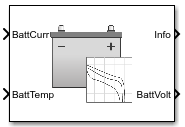Documentation

### This is machine translation

Mouseover text to see original. Click the button below to return to the English version of the page.

# Datasheet Battery

• Library:
• Powertrain Blockset / Energy Storage and Auxiliary Drive / Datasheet Battery## Description

The Datasheet Battery block implements a lithium-ion, lithium-polymer, or lead-acid battery that you can parameterize using manufacturer data. To create the open-circuit voltage and internal resistance parameters that you need for the block, use the manufacturer discharge characteristics by temperature data. For an example, see Generate Parameter Data for Datasheet Battery Block.

To determine the battery output voltage, the block uses lookup tables for the battery open-circuit voltage and the internal resistance. The lookup tables are functions of the state-of charge (SOC) and battery temperature, characterizing the battery performance at various operating points:

`$\begin{array}{l}{E}_{m}=f\left(SOC\right)\\ {R}_{int}=f\left(T,SOC\right)\end{array}$`

To calculate the voltage, the block implements these equations.

Positive current indicates battery discharge. Negative current indicates battery charge.

### Power Accounting

For the power accounting, the block implements these equations.

Bus Signal DescriptionEquations

`PwrInfo`

`PwrTrnsfrd` — Power transferred between blocks

• Positive signals indicate flow into block

• Negative signals indicate flow out of block

`PwrLdBatt`

Battery network power

`PwrNotTrnsfrd` — Power crossing the block boundary, but not transferred

• Positive signals indicate an input

• Negative signals indicate a loss

`PwrLossBatt`

Battery network power loss

${P}_{LossBatt}=-{N}_{p}{N}_{s}{I}_{batt}{}^{2}{R}_{int}$

`PwrStored` — Stored energy rate of change

• Positive signals indicate an increase

• Negative signals indicate a decrease

`PwrStoredBatt`

Battery network power stored

${P}_{StoredBatt}={P}_{Batt}+{P}_{LossBatt}$

The equations use these variables.

 SOC State-of-charge Em Battery open-circuit voltage Ibatt Per module battery current PLdBatt Battery network power Pbatt Battery power PLossBatt Battery network power loss PStoredBatt Battery network power stored Iin Combined current flowing from the battery network Rint Battery internal resistance Ns Number of cells in series Np Number of cells in parallel Vout, Vbatt Combined voltage of the battery network VT Per module battery voltage Capbatt Battery capacity LdAmpHr Battery energy

## Ports

### Inputs

expand all

Rated battery capacity at the nominal temperature, Capbatt, in Ah.

#### Dependencies

To create this port, select ```External Input``` for the Initial battery capacity parameter.

Combined current flowing from the battery network, Iin, in A.

Temperature measured at the battery housing, T, in K.

### Output

expand all

Bus signal containing these block calculations.

SignalDescriptionVariableUnits

`BattCurr`

Combined current flowing from the battery network

Ibatt

A

`BattAmpHr`

Battery energy

LdAmpHr

A*h

`BattSoc`

State-of-charge capacity

SOC

NA

`BattVolt`

Combined voltage of the battery network

VoutV

`BattPwr`

Battery network power

Pbatt

W

`PwrInfo`

`PwrTrnsfrd`

`PwrLdBatt`

Battery network power

PLdBatt

W

`PwrNotTrnsfrd`

`PwrLossBatt`

Battery network power loss

PLossBatt

W

`PwrStored`

`PwrStoredBatt`

Battery network power stored

PStoredBatt

W

Combined voltage of the battery network, Vout, in V.

## Parameters

expand all

#### Block Options

Initial battery capacity, Capbatt, in Ah.

#### Dependencies

Block Parameter Initial battery capacity Option

Creates

`External Input`

Input port `CapInit`
`Parameter`Parameter Initial battery capacity, BattCapInit

Select `Filtered` to apply a first-order filter to the output batter voltage.

#### Dependencies

Setting Output battery voltage parameter to `Filtered` creates these parameters:

• Output battery voltage time constant, Tc

• Output battery voltage initial value, Vinit

Rated battery capacity at the nominal temperature, in Ah.

Open-circuit voltage data curve, Em, as a function of the discharged capacity for `P` operating points, in V.

Discharge capacity breakpoints for `P` operating points, dimensionless.

Although this parameter is the same as the Battery capacity breakpoints 2, CapSOCBp parameter, the block uses unique parameters for calibration flexibility.

Internal resistance map, Rint, as a function of `N` temperatures and `M` SOCs, in ohms.

Battery temperature breakpoints for `N` temperatures, in K.

Battery capacity breakpoints for `M` SOCs, dimensionless.

Although this parameter is the same as the Open circuit voltage breakpoints 1, CapLUTBp parameter, the block uses unique parameters for calibration flexibility.

Number of cells in series, dimensionless, Ns.

Number of cells in parallel, dimensionless, Np.

Initial battery capacity, Capbatt, in Ah.

#### Dependencies

Block Parameter Initial battery capacity Option

Creates

`External Input`

Input port `CapInit`
`Parameter`Parameter Initial battery capacity, BattCapInit

Output battery voltage time constant, Tc, in s. Used in a first-order voltage filter.

#### Dependencies

Setting Output battery voltage parameter to `Filtered` creates these parameters:

• Output battery voltage time constant, Tc

• Output battery voltage initial value, Vinit

Output battery voltage initial value, Vinit, in V. Used in a first-order voltage filter.

#### Dependencies

Setting Output battery voltage parameter to `Filtered` creates these parameters:

• Output battery voltage time constant, Tc

• Output battery voltage initial value, Vinit

 Arrhenius, S.A. “Über die Dissociationswärme und den Einflusß der Temperatur auf den Dissociationsgrad der Elektrolyte.” Journal of Physical Chemistry. 4 (1889): 96–116.

 Connors, K. Chemical Kinetics. New York: VCH Publishers, 1990.

 Ji, Yan, Yancheng Zhang, and Chao-Yang Wang. Journal of the Electrochemical Society. Volume 160, Issue 4 (2013), A636-A649.

##### Support평가판 신청